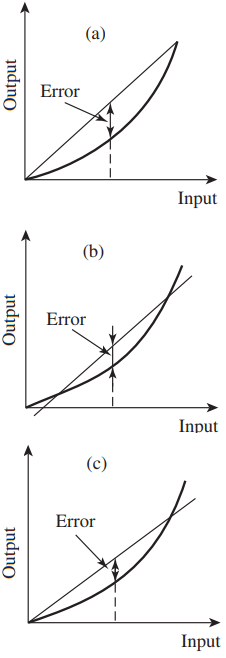# Characteristics of Sensors

There are a number of parameters that characterize sensors’ performance. The time-independent characteristics are called the static characteristics, while the time-dependent characteristics are called the dynamic characteristics.

The static characteristics characterize the sensor output after it has settled due to changes in the physical quantity being measured. The dynamic characteristics describe the sensor characteristics from the time the physical quantity has changed to the time before the output has settled.

# Static Characteristics of Sensors

Range: Minimum to maximum value that can be measured is the range. The range defines the allowable range of the physical quantity that can be detected by the sensor.

Accuracy: The difference between true and actual measured value is the accuracy. It is commonly expressed as a percentage of full-scale value.

For example, if a temperature sensor has a range of 0 to 200°C and an accuracy of 0.5% full-scale value, then the temperature read by the sensor is off from the true actual temperature by 1°. Note that the accuracy error can be improved by calibration.

Sensitivity: The relationship between the measured input and the output of the sensor is its sensitivity. If the sensor has a linear input–output relationship, then the sensitivity is the slope of this curve.

Sometimes, this parameter is used to indicate the sensitivity of the sensor to non-measured input (response due to transverse motion when the sensor is designed to measure axial motion) or the environment (temperature).

Resolution: The smallest change in input value that will produce an observable change in the output is the resolution. The inherent resolution should be distinguished from the display device resolution.

Hysteresis: The maximum difference in sensor output for the same input quantity is the hysteresis, with one measurement taken while the input was increasing from zero and the other by decreasing the input from the maximum input.

A sensor with hysteresis will have a different output value that is a function of whether the input quantity was increasing or decreasing when the measurement was made. The hysteresis error is illustrated in Figure 1.

Repeatability: Error in output value for repeated application of the same input value is called repeatability or precision. The smaller the repeatability error, the higher the measurement precision would be.

Repeatability is affected by signal interference, vibration, and temperature fluctuation. Repeatability error cannot be reduced by calibration.

Non-Linearity Error: Most sensors are designed to have a linear output, but their output is not perfectly linear. The non-linearity error is a measure of the maximum difference between the sensor actual output and a straight line fit to the sensor input–output data and is usually specified as a percentage of the full-scale output. There is no unique way to obtain the straight line fit.

The straight line can connect the minimum and maximum output values that define the sensor range, or it can be obtained from a least-square fit to the entire input–output data or from a least-square fit to the input–output data with one end of the line passing through the origin.Figure 2 nonlinearity error. (a) Minimum to maximum fit; (b) Least-square fitthrough data; (c) Least-square fit with one end through the origin.

Figure 2 illustrates these cases and shows that the magnitude of this error is dependent on how this error is defined.

Stability: Stability or drift refers to the variation of the output with time when the input quantity is not changing. When no input is applied to the sensor, the output variation is called the zero drift. Stability affects the repeatability of the measurement.

## Dynamic Characteristics of Sensors

Rise Time: The time it takes the output to change a certain percentage is the rise time. A common measure is the time for the output to change from 10 to 90% of the final steady–state value.

Time Constant: This is defined as the time it takes the output to reach 63.2% of the final output. A large time constant implies a sluggish sensor, while one with a small value indicates a rapidly responding sensor.

If the sensor has first-order response characteristics, then it takes about four time constants to reach the final value when subjected to a step input.

Settling Time: The time it takes the output to reach within certain percentage of the final steady–state value is the settling time. A common value is the 2% settling time.

The rise time, time constant, and settling time are illustrated in Figure 3 for a sensor with first-order response characteristics.